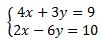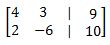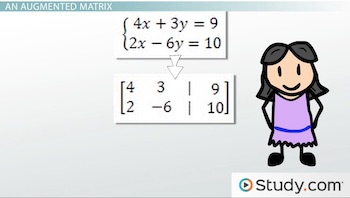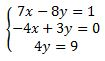# How to Write an Augmented Matrix for a Linear System

An error occurred trying to load this video.

Try refreshing the page, or contact customer support.

Coming up next: How to Perform Matrix Row Operations

### You're on a roll. Keep up the good work!

Replay
Your next lesson will play in 10 seconds
• 0:02 A Linear System
• 0:39 An Augmented Matrix
• 1:40 Example 1
• 2:53 Example 2
• 3:22 Lesson Summary

Want to watch this again later?

Timeline
Autoplay
Autoplay
Speed

#### Recommended Lessons and Courses for You

Lesson Transcript
Instructor: Yuanxin (Amy) Yang Alcocer

Amy has a master's degree in secondary education and has taught math at a public charter high school.

Watch this video lesson to learn how you can turn your system of equations into matrix form. The matrix form is another way of writing your linear system that is sometimes easier to work with.

## A Linear System

In this video lesson, we talk about the linear system, a collection of linear equations. Recall that a linear equation is an equation of degree one with only two variables, usually x and y. Our system, then, is a collection of two or more of these linear equations. We usually see our linear systems written as a list of equations with a bracket in front of them, like this:This is the most common way you will see linear systems written. Notice that we have our x terms first, followed by our y terms, then an equals sign, and finally our constant.

## An Augmented Matrix

The other form in which we can write our linear systems is called an augmented matrix, which is a combination of two matrices. If you look at just the coefficients of our linear system along with their sign, then you can split our linear system into one matrix on the left side of the equation and another matrix on the other side of the equation. Combining these two matrices then gives us our augmented matrix. We use a vertical line to note where our equals sign is that splits the matrix. So our augmented matrix looks like this:We have only our coefficients listed. Our coefficients are organized into their appropriate columns. All the x term coefficients are in the first column, all the y term coefficients are in the second column, and all our constants are in the final column. The vertical lines show you where the equals sign is. We can easily write our linear equations directly on top of our matrix numbers, and you can see how the numbers line up nicely with each other:## Example 1

Writing an augmented matrix from a linear system is easy. First, you organize your linear equations so that your x terms are first, followed by your y terms, then your equals sign, and finally your constant. Once you have all your equations in this format, all it takes for you to turn it into an augmented matrix is to write your coefficients down along with their positive or negative sign. For the equals sign, you use a vertical line.

Let's look at how to turn this linear system into an augmented matrix.To unlock this lesson you must be a Study.com Member.

### Register to view this lesson

Are you a student or a teacher?

### Unlock Your Education

#### See for yourself why 30 million people use Study.com

##### Become a Study.com member and start learning now.
Back
What teachers are saying about Study.com

### Earning College Credit

Did you know… We have over 160 college courses that prepare you to earn credit by exam that is accepted by over 1,500 colleges and universities. You can test out of the first two years of college and save thousands off your degree. Anyone can earn credit-by-exam regardless of age or education level.